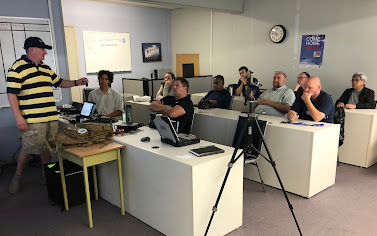## SARC Events

 FoxHunt Video
 SARC Courses Course Information
 Field Day Video

## Information and examples to make practical single-layer air-core coils.

Whenever you are working on an RF filter, choke, antenna or an oscillator design, most likely you will need a coil. Under some circumstances, the coil might not be available commercially or may be expensive. If this is the case, you have to make your own. This article will provide the information and examples to make practical single-layer air-core coils.

The well known Wheeler’s formula is used to calculate the approximate inductance of a single-layer air-core coil.  In 1925, Harold A. Wheeler published his formula as shown below. This is not a theoretical formula but an empirical one and is accurate to 3~4%.

Where:
L: inductance (μH)
d: diameter of coil (inch)
n: turns of winding
l: length of coil (inch)

For best result by using this formula, the length of the coil (l) should be equal to or greater than 40% (0.4) of the coil diameter (d). If you study the formula carefully, you will find that the inductance is proportional to the square of the turns. That is, if you want to double the coil’s inductance, you don’t have to double the turns, you just add 40% more turns to the coil. For example, if you have a coil of 47μH and the winding has 100 turns, and you want to double the coil’s inductance to 94μH, you simply add another 40 turns to it, for a total of 140 turns.

Example 1: What is the inductance of a coil if the coil has 86 turns wound on a 1.25 inch diameter round form, and the coil’s length is 1.5 inches? In this case, d = 1.25,  l = 1.5 and n = 86.

Since the input data are only good to (at most) 3 significant figures, the result is only good to 3 figures so you would round the answer to 140µH.  To calculate the number of turns of a single-layer air-core coil for a given value of inductance, re-arrange the formula and it becomes:

Example 2: To build an AM radio, an inductance of 260μH is required. The form on which the coil is to be wound has a diameter of two inches and one inch is chosen to be the length of that coil. Then d = 2 inches, l = 1 inch and L = 260.

Since the coil is 1 inch long, the number of turns per inch is 70 / 1 = 70. Consulting the chart at the end of this article, we find that 28 AWG enameled wire can be used.

To make it easy for you to build your single-layer air-core coil, the author has written a script with PHP to do all the calculations for you. All you have to do is just plug in the desired inductance, the diameter of the coil form, the wire gauge and, if wanted, the operating frequency (for the Q or quality factor of the coil). Then you will be given the number of turns of the coil, the length of the coil and the length of wire needed. Since you know the length of the coil, you just wind the coil tightly to that length, which saves you from having to count the turns.  I hate to do the counting because it is tedious and frustrating when you lose count, believe me. You can fool around with the diameter of the coil form and/or the wire gauge to optimize your coil.

I’ve placed the script on our website.  You can try it out from the link below.The above picture shows the final product of the example 2 in this article.
 The coil is wound with 28 AWG enameled wire on a 2 inch diameter pill bottle.  254μH is measured, which is 2.3% less than the target value 260μH.  This result is more than adequate for most applications.

Have fun on winding and I hope this has been useful.

~ Hiu VE7YXG